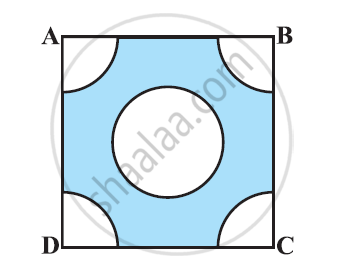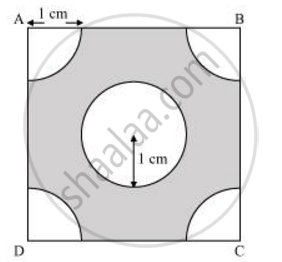Share

# From each corner of a square of side 4 cm a quadrant of a circle of radius 1 cm is cut and also a circle of diameter 2 cm is cut as shown in the given figure. Find the area of the remaining portion of the square - CBSE Class 10 - Mathematics

ConceptAreas of Combinations of Plane Figures

#### Question

From each corner of a square of side 4 cm a quadrant of a circle of radius 1 cm is cut and also a circle of diameter 2 cm is cut as shown in the given figure. Find the area of the remaining portion of the square [Use Π = 22/7]#### SolutionEach quadrant is a sector of 90° in a circle of 1 cm radius.

Area of each quadrant = 90^@/360^@pir^2

= 1/4xx22/7xx(1)^2 = 22/28 cm^2

Area of square = (Side)2 = (4)2 = 16 cm2

Area of circle = πr2 = π (1)2

= 22/7 cm^2

Area of the shaded region = Area of square − Area of circle − 4 × Area of quadrant

 = 16   -22/7 - 4 xx 22/28

= 16-22/7-22/7 = 16 - 44/7

= (112-44)/7 = 68/7 cm^2

Is there an error in this question or solution?

#### APPEARS IN

NCERT Solution for Mathematics Textbook for Class 10 (2019 to Current)
Chapter 12: Areas Related to Circles
Ex. 12.30 | Q: 5 | Page no. 235
Solution From each corner of a square of side 4 cm a quadrant of a circle of radius 1 cm is cut and also a circle of diameter 2 cm is cut as shown in the given figure. Find the area of the remaining portion of the square Concept: Areas of Combinations of Plane Figures.
S